# Selina Solutions Concise Maths Class 10 Chapter 1 [Goods and Services Tax] Exercise 1(B)

While paying tax on sale of goods and services, you can avail the tax you have already paid on the purchase of the above goods/service and pay only the balance amount as tax. This is called Input Tax Credit (ITC). Students will encounter problems based on this concept in this exercise. The solutions for all these problems are available in Selina Solutions for Class 10 Maths. Students willing to clear doubts on solving any problem of this exercise can download the Concise Selina Solutions for Class 10 Maths Chapter 1 GST [Goods and Services Tax] Exercise 1(B) PDF which is given below.

## Selina Solutions Concise Maths Class 10 Chapter 1 GST [Goods and Services Tax] Exercise 1(B) Download PDF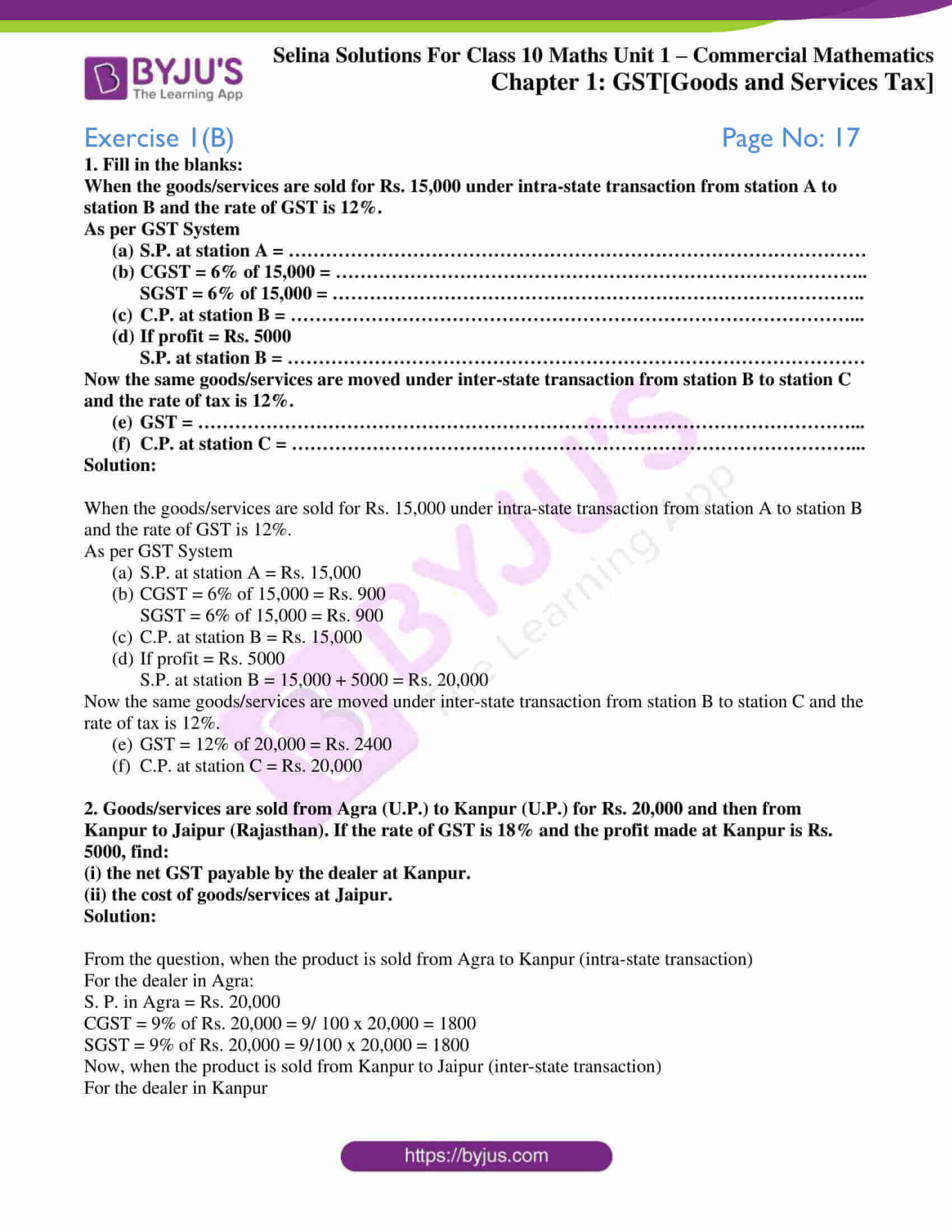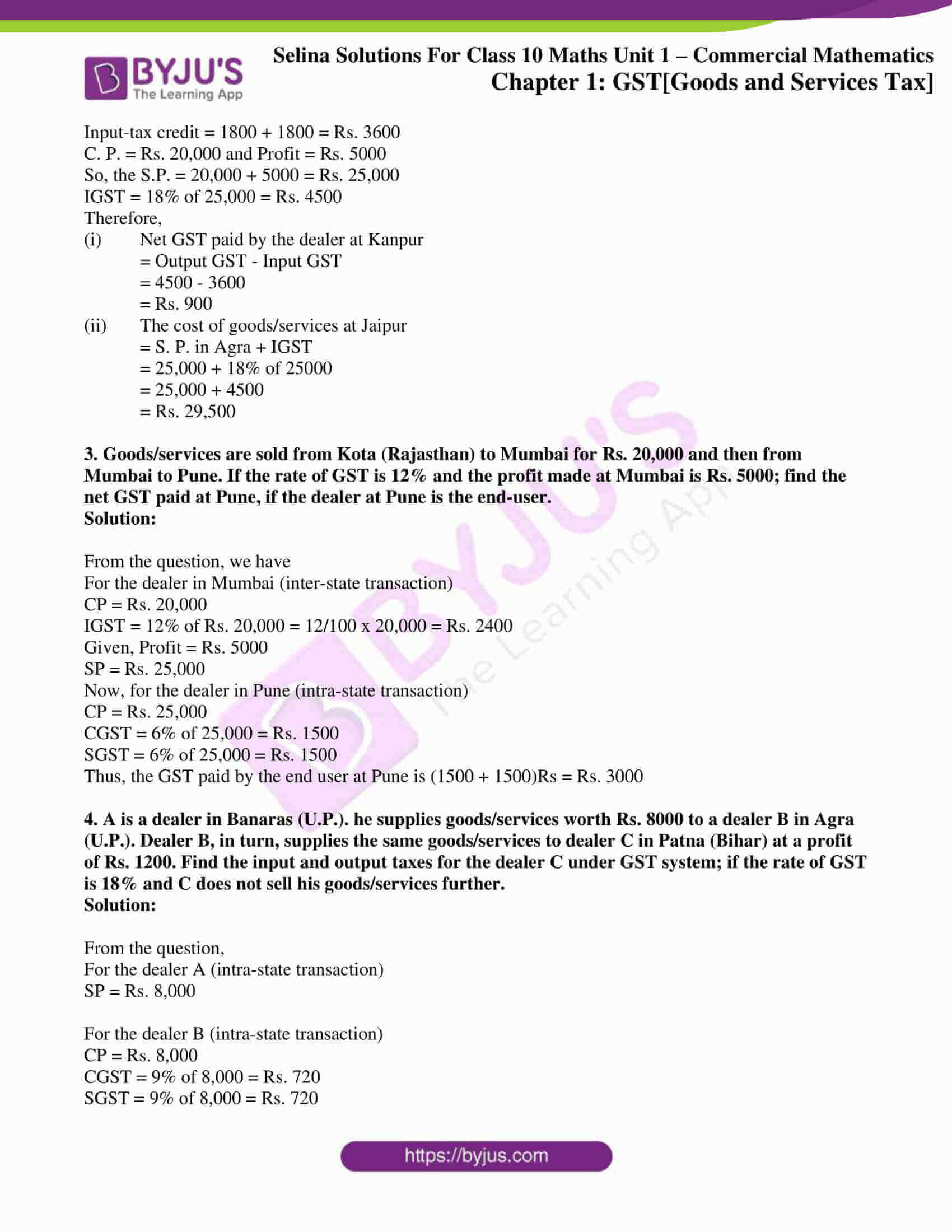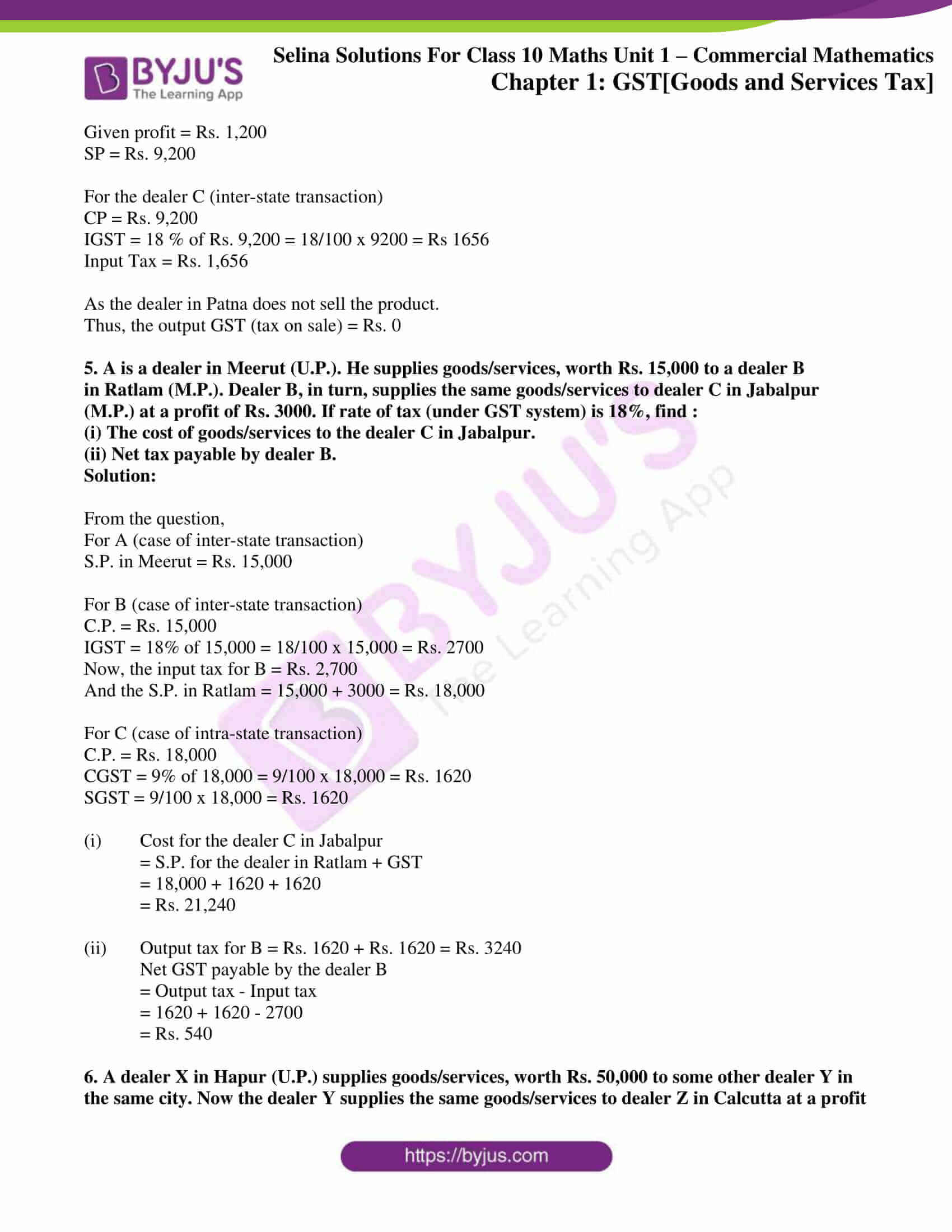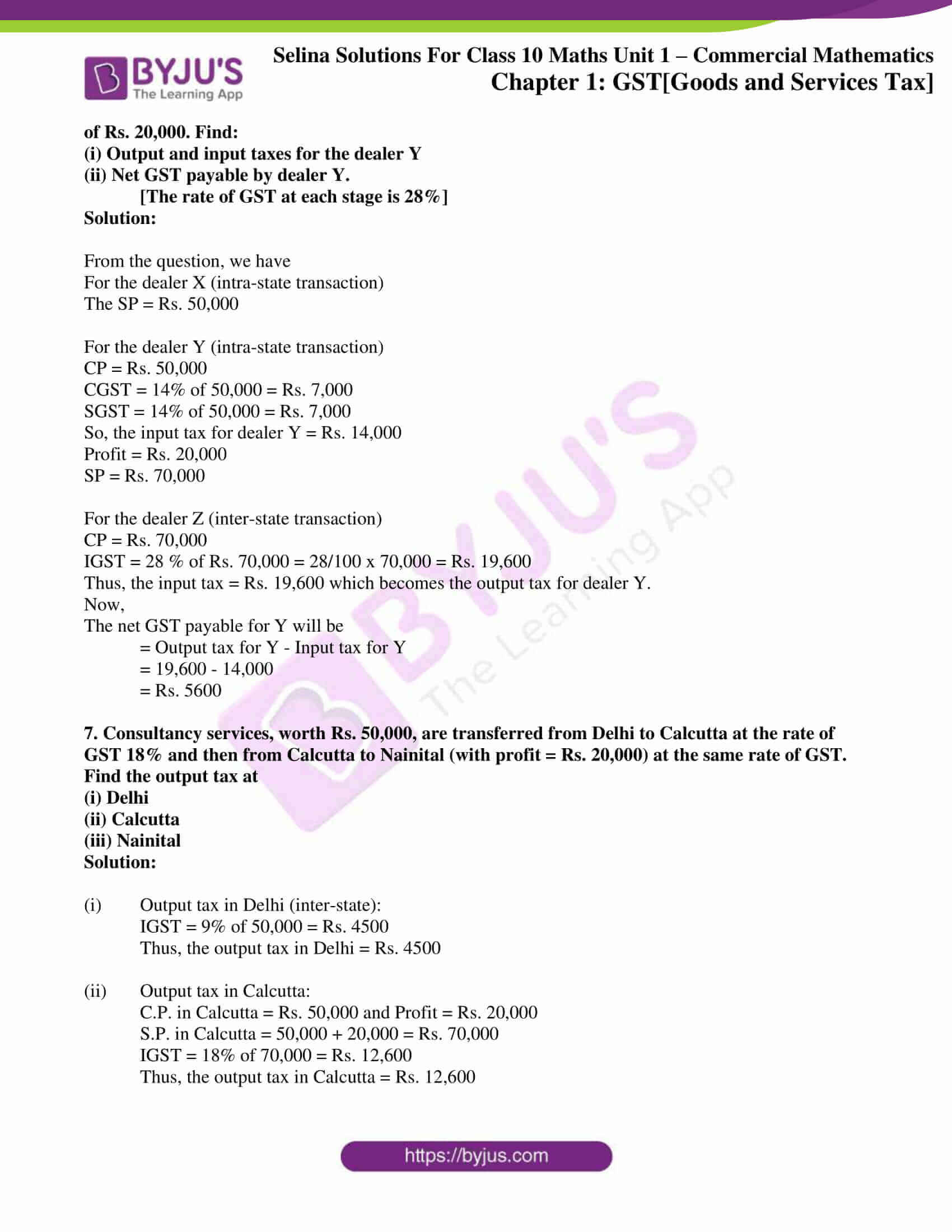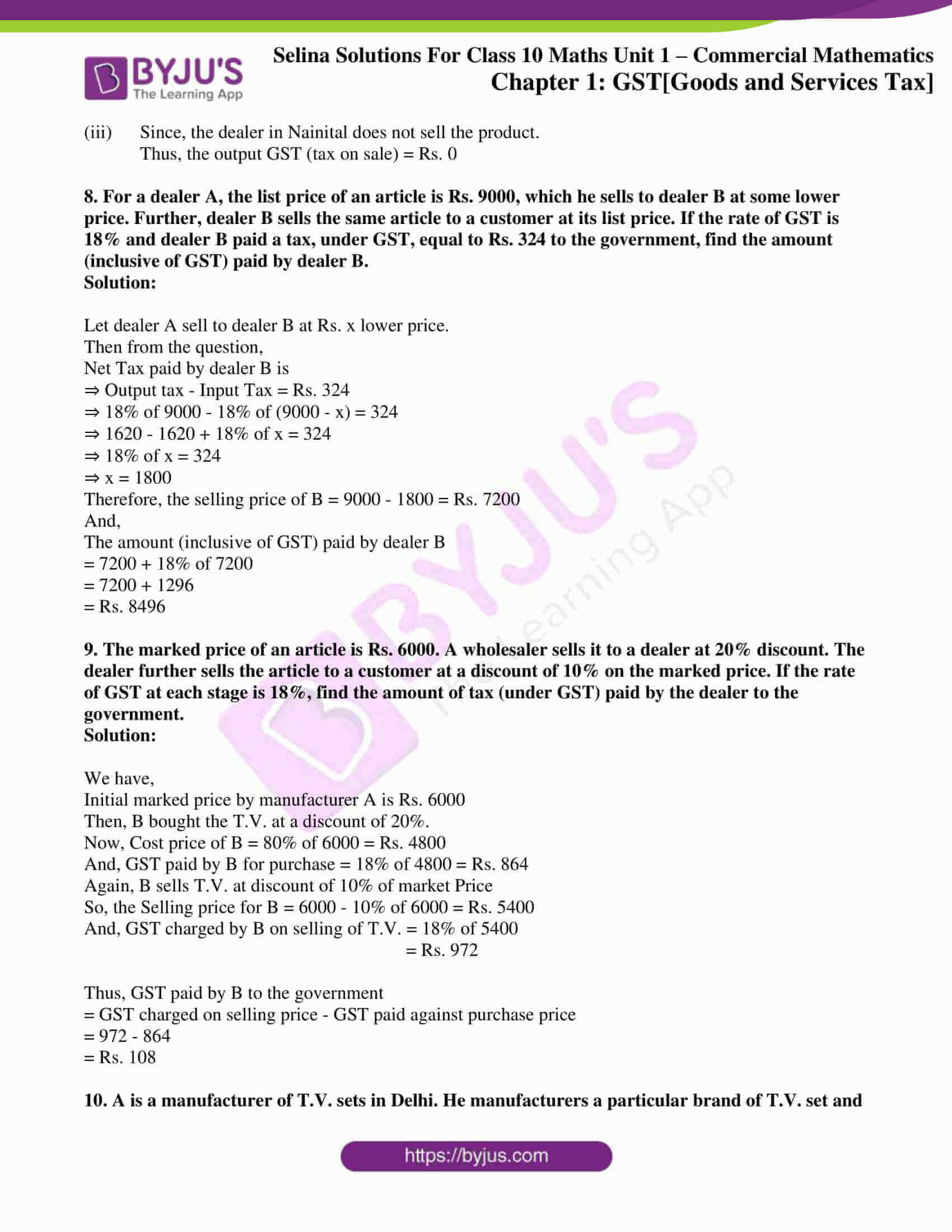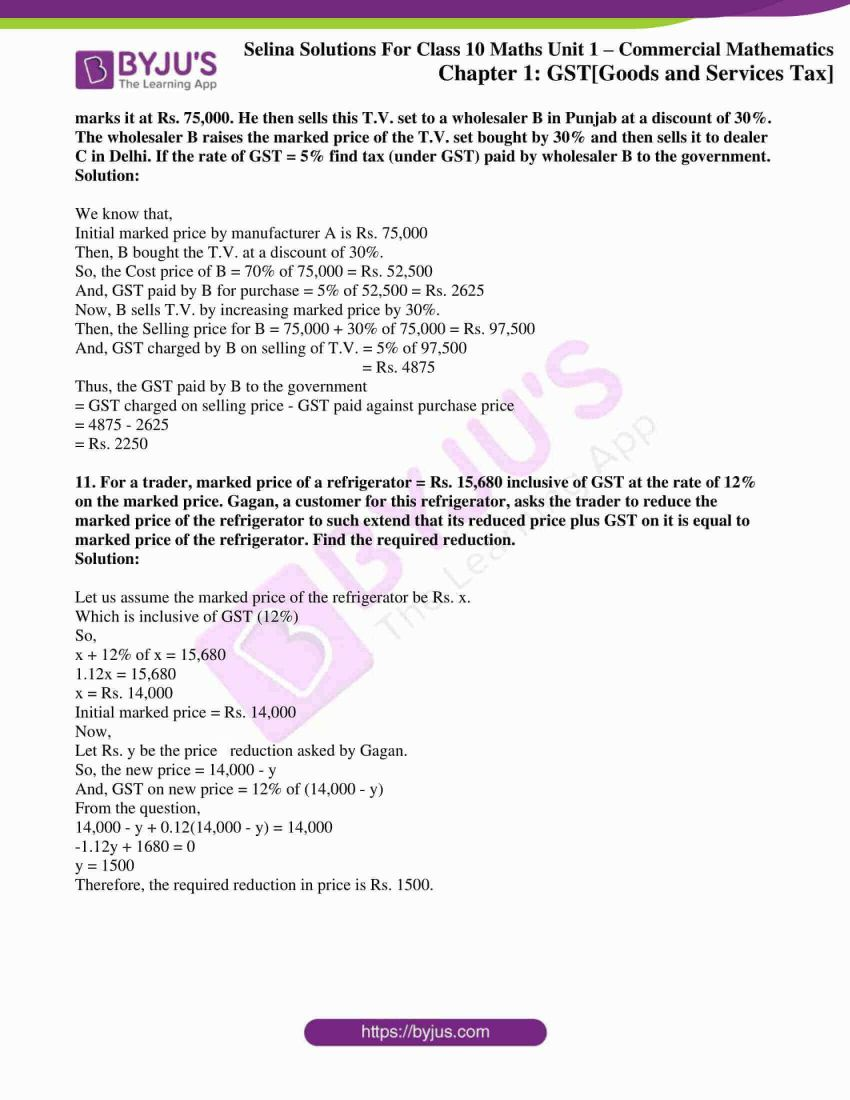### Access another exercise of Selina Solutions Concise Maths Class 10 Chapter 1 [Goods and Services Tax]

Exercise 1(A) Solutions

### Exercise 1(B) Page No: 17

1. Fill in the blanks:

When the goods/services are sold for Rs. 15,000 under intra-state transaction from station A to station B and the rate of GST is 12%.

As per GST System

(a) S.P. at station A = …………………………………………………………………………………

(b) CGST = 6% of 15,000 = …………………………………………………………………………..
SGST = 6% of 15,000 = …………………………………………………………………………..

(c) C.P. at station B = …………………………………………………………………………………

(d) If profit = Rs. 5000
S.P. at station B = …………………………………………………………………………………

Now the same goods/services are moved under inter-state transaction from station B to station C and the rate of tax is 12%.

(e) GST = ………………………………………………………………………………………………

(f) C.P. at station C = …………………………………………………………………………………

Solution:

When the goods/services are sold for Rs. 15,000 under intra-state transaction from station A to station B and the rate of GST is 12%.

As per GST System

(a) S.P. at station A = Rs. 15,000

(b) CGST = 6% of 15,000 = Rs. 900

SGST = 6% of 15,000 = Rs. 900

(c) C.P. at station B = Rs. 15,000

(d) If profit = Rs. 5000

S.P. at station B = 15,000 + 5000 = Rs. 20,000

Now the same goods/services are moved under inter-state transaction from station B to station C and the rate of tax is 12%.

(e) GST = 12% of 20,000 = Rs. 2400

(f) C.P. at station C = Rs. 20,000

2. Goods/services are sold from Agra (U.P.) to Kanpur (U.P.) for Rs. 20,000 and then from Kanpur to Jaipur (Rajasthan). If the rate of GST is 18% and the profit made at Kanpur is Rs. 5000, find:

(i) the net GST payable by the dealer at Kanpur.

(ii) the cost of goods/services at Jaipur.

Solution:

From the question, when the product is sold from Agra to Kanpur (intra-state transaction)

For the dealer in Agra:

S. P. in Agra = Rs. 20,000

CGST = 9% of Rs. 20,000 = 9/ 100 x 20,000 = 1800

SGST = 9% of Rs. 20,000 = 9/100 x 20,000 = 1800

Now, when the product is sold from Kanpur to Jaipur (inter-state transaction)

For the dealer in Kanpur

Input-tax credit = 1800 + 1800 = Rs. 3600

C. P. = Rs. 20,000 and Profit = Rs. 5000

So, the S.P. = 20,000 + 5000 = Rs. 25,000

IGST = 18% of 25,000 = Rs. 4500

Therefore,

(i) Net GST paid by the dealer at Kanpur

= Output GST – Input GST

= 4500 – 3600

= Rs. 900

(ii) The cost of goods/services at Jaipur

= S. P. in Agra + IGST

= 25,000 + 18% of 25000

= 25,000 + 4500

= Rs. 29,500

3. Goods/services are sold from Kota (Rajasthan) to Mumbai for Rs. 20,000 and then from Mumbai to Pune. If the rate of GST is 12% and the profit made at Mumbai is Rs. 5000; find the net GST paid at Pune, if the dealer at Pune is the end-user.

Solution:

From the question, we have

For the dealer in Mumbai (inter-state transaction)

CP = Rs. 20,000

IGST = 12% of Rs. 20,000 = 12/100 x 20,000 = Rs. 2400

Given, Profit = Rs. 5000

SP = Rs. 25,000

Now, for the dealer in Pune (intra-state transaction)

CP = Rs. 25,000

CGST = 6% of 25,000 = Rs. 1500

SGST = 6% of 25,000 = Rs. 1500

Thus, the GST paid by the end user at Pune is (1500 + 1500)Rs = Rs. 3000

4. A is a dealer in Banaras (U.P.). he supplies goods/services worth Rs. 8000 to a dealer B in Agra (U.P.). Dealer B, in turn, supplies the same goods/services to dealer C in Patna (Bihar) at a profit of Rs. 1200. Find the input and output taxes for the dealer C under GST system; if the rate of GST is 18% and C does not sell his goods/services further.

Solution:

From the question,

For the dealer A (intra-state transaction)

SP = Rs. 8,000

For the dealer B (intra-state transaction)

CP = Rs. 8,000

CGST = 9% of 8,000 = Rs. 720

SGST = 9% of 8,000 = Rs. 720

Given profit = Rs. 1,200

SP = Rs. 9,200

For the dealer C (inter-state transaction)

CP = Rs. 9,200

IGST = 18 % of Rs. 9,200 = 18/100 x 9200 = Rs 1656

Input Tax = Rs. 1,656

As the dealer in Patna does not sell the product.

Thus, the output GST (tax on sale) = Rs. 0

5. A is a dealer in Meerut (U.P.). He supplies goods/services, worth Rs. 15,000 to a dealer B in Ratlam (M.P.). Dealer B, in turn, supplies the same goods/services to dealer C in Jabalpur (M.P.) at a profit of Rs. 3000. If rate of tax (under GST system) is 18%, find :

(i) The cost of goods/services to the dealer C in Jabalpur.

(ii) Net tax payable by dealer B.

Solution:

From the question,

For A (case of inter-state transaction)

S.P. in Meerut = Rs. 15,000

For B (case of inter-state transaction)

C.P. = Rs. 15,000

IGST = 18% of 15,000 = 18/100 x 15,000 = Rs. 2700

Now, the input tax for B = Rs. 2,700

And the S.P. in Ratlam = 15,000 + 3000 = Rs. 18,000

For C (case of intra-state transaction)

C.P. = Rs. 18,000

CGST = 9% of 18,000 = 9/100 x 18,000 = Rs. 1620

SGST = 9/100 x 18,000 = Rs. 1620

(i) Cost for the dealer C in Jabalpur

= S.P. for the dealer in Ratlam + GST

= 18,000 + 1620 + 1620

= Rs. 21,240

(ii) Output tax for B = Rs. 1620 + Rs. 1620 = Rs. 3240

Net GST payable by the dealer B

= Output tax – Input tax

= 1620 + 1620 – 2700

= Rs. 540

6. A dealer X in Hapur (U.P.) supplies goods/services, worth Rs. 50,000 to some other dealer Y in the same city. Now the dealer Y supplies the same goods/services to dealer Z in Calcutta at a profit of Rs. 20,000. Find:

(i) Output and input taxes for the dealer Y

(ii) Net GST payable by dealer Y.

[The rate of GST at each stage is 28%]

Solution:

From the question, we have

For the dealer X (intra-state transaction)

The SP = Rs. 50,000

For the dealer Y (intra-state transaction)

CP = Rs. 50,000

CGST = 14% of 50,000 = Rs. 7,000

SGST = 14% of 50,000 = Rs. 7,000

So, the input tax for dealer Y = Rs. 14,000

Profit = Rs. 20,000

SP = Rs. 70,000

For the dealer Z (inter-state transaction)

CP = Rs. 70,000

IGST = 28 % of Rs. 70,000 = 28/100 x 70,000 = Rs. 19,600

Thus, the input tax = Rs. 19,600 which becomes the output tax for dealer Y.

Now,

The net GST payable for Y will be

= Output tax for Y – Input tax for Y

= 19,600 – 14,000

= Rs. 5600

7. Consultancy services, worth Rs. 50,000, are transferred from Delhi to Calcutta at the rate of GST 18% and then from Calcutta to Nainital (with profit = Rs. 20,000) at the same rate of GST. Find the output tax at

(i) Delhi

(ii) Calcutta

(iii) Nainital

Solution:

(i) Output tax in Delhi (inter-state):

IGST = 9% of 50,000 = Rs. 4500

Thus, the output tax in Delhi = Rs. 4500

(ii) Output tax in Calcutta:

C.P. in Calcutta = Rs. 50,000 and Profit = Rs. 20,000

S.P. in Calcutta = 50,000 + 20,000 = Rs. 70,000

IGST = 18% of 70,000 = Rs. 12,600

Thus, the output tax in Calcutta = Rs. 12,600

(iii) Since, the dealer in Nainital does not sell the product.

Thus, the output GST (tax on sale) = Rs. 0

8. For a dealer A, the list price of an article is Rs. 9000, which he sells to dealer B at some lower price. Further, dealer B sells the same article to a customer at its list price. If the rate of GST is 18% and dealer B paid a tax, under GST, equal to Rs. 324 to the government, find the amount (inclusive of GST) paid by dealer B.

Solution:

Let dealer A sell to dealer B at Rs. x lower price.

Then from the question,

Net Tax paid by dealer B is

⇒ Output tax – Input Tax = Rs. 324

⇒ 18% of 9000 – 18% of (9000 – x) = 324

⇒ 1620 – 1620 + 18% of x = 324

⇒ 18% of x = 324

⇒ x = 1800

Therefore, the selling price of B = 9000 – 1800 = Rs. 7200

And,

The amount (inclusive of GST) paid by dealer B

= 7200 + 18% of 7200

= 7200 + 1296

= Rs. 8496

9. The marked price of an article is Rs. 6000. A wholesaler sells it to a dealer at 20% discount. The dealer further sells the article to a customer at a discount of 10% on the marked price. If the rate of GST at each stage is 18%, find the amount of tax (under GST) paid by the dealer to the government.

Solution:

We have,

Initial marked price by manufacturer A is Rs. 6000

Then, B bought the T.V. at a discount of 20%.

Now, Cost price of B = 80% of 6000 = Rs. 4800

And, GST paid by B for purchase = 18% of 4800 = Rs. 864

Again, B sells T.V. at discount of 10% of market Price

So, the Selling price for B = 6000 – 10% of 6000 = Rs. 5400

And, GST charged by B on selling of T.V. = 18% of 5400

= Rs. 972

Thus, GST paid by B to the government

= GST charged on selling price – GST paid against purchase price

= 972 – 864

= Rs. 108

10. A is a manufacturer of T.V. sets in Delhi. He manufacturers a particular brand of T.V. set and marks it at Rs. 75,000. He then sells this T.V. set to a wholesaler B in Punjab at a discount of 30%. The wholesaler B raises the marked price of the T.V. set bought by 30% and then sells it to dealer C in Delhi. If the rate of GST = 5% find tax (under GST) paid by wholesaler B to the government.

Solution:

We know that,

Initial marked price by manufacturer A is Rs. 75,000

Then, B bought the T.V. at a discount of 30%.

So, the Cost price of B = 70% of 75,000 = Rs. 52,500

And, GST paid by B for purchase = 5% of 52,500 = Rs. 2625

Now, B sells T.V. by increasing marked price by 30%.

Then, the Selling price for B = 75,000 + 30% of 75,000 = Rs. 97,500

And, GST charged by B on selling of T.V. = 5% of 97,500

= Rs. 4875

Thus, the GST paid by B to the government

= GST charged on selling price – GST paid against purchase price

= 4875 – 2625

= Rs. 2250

11. For a trader, marked price of a refrigerator = Rs. 15,680 inclusive of GST at the rate of 12% on the marked price. Gagan, a customer for this refrigerator, asks the trader to reduce the marked price of the refrigerator to such extend that its reduced price plus GST on it is equal to marked price of the refrigerator. Find the required reduction.

Solution:

Let us assume the marked price of the refrigerator be Rs. x.

Which is inclusive of GST (12%)

So,

x + 12% of x = 15,680

1.12x = 15,680

x = Rs. 14,000

Initial marked price = Rs. 14,000

Now,

Let Rs. y be the price reduction asked by Gagan.

So, the new price = 14,000 – y

And, GST on new price = 12% of (14,000 – y)

From the question,

14,000 – y + 0.12(14,000 – y) = 14,000

-1.12y + 1680 = 0

y = 1500

Therefore, the required reduction in price is Rs. 1500.